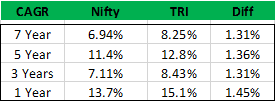# What is total Return Index index and how is it calculated?

I was reading that DSP BlackRock will be bench marking their funds against the total Return Index index (TRI), could someone give me a low down on the TRI. And also how will this impact MF returns calculations.

1 Like

1 Like

As we know Nifty index represents a basket of 50 stocks. The increase or decrease in the index value gives a rough estimate of the overall capital appreciation in stocks. For this reasons, Nifty is often used as a benchmark for market performance. However, Nifty captures only the capital appreciation from equity investments and does not consider the dividend receipts. This is where Total Return Index comes into play.

The ‘Total Return Index’ of Nifty besides capturing the capital appreciation of Nifty, also captures the effect of dividend received. So think of the Total Return Index as Nifty plus the dividends received.

The difference in the ‘Compounded average growth rate’ (CAGR) between Nifty 50 and ‘Total Return Index’ of Nifty averages around 1.35%. The details are as follows -While the percentage difference of 1.35% seems small, do remember this is a compounded return, and it does make a significant difference over a long investment period. To give you a perspective, if you choose to invest a sum of Rs.500,000/- in an instrument which returns 12% CAGR, then at the end of 10 years you will have Rs.1,552,924/- with you. However, if we add the 1.35% dividend receipt to this, then at 13.5% CAGR, Rs.500,000/- would grow to Rs.1,789,589/-.

The 1.35% extra return gives you an additional Rs.236,665/- over the 10 year investment period!

3 Likes

From February 1 , mutual fund schemes are mandated by Sebi to use Total Return Index or TRI to benchmark their performance.

2 Likes